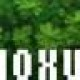# Samiam’s Scribble Pad

## January 31, 2009

### Intersection of a line and a Cone

Filed under: Uncategorized — admin @ 11:12 am

Currently I am trying to make some volume lights at work

To optimise I need to work out where the eye ray intersects a implicit cone

You will find some links and a quick animation below

When I have cracked the renderman solution I may post that too

With a bit of googling I managed to understand the problem, although it is probably in my calculus book too

here is how I sum it up:

The intersection of a line and a cone, can be dervied by creating a plane at the origin on the cone whose normal is orthogonal to both the cone vector and the line, the plane passes through the point at the tip of the cone, this will intersect with the cone as zero, one or two lines, depending on the angle between the plane and the cone axis, either greater than, equal to or less than the cone angle, where these line intersect the original line is where the intersection points are.

To solve you simply measure the angles between the various vectors and then solve the intersection of the lines

Here are some links

A PDF from geometric tools

A Grid of all intersection

As well as a book excerpt from a book I dont have “Ching-Kuang Shene, Computing the Intersection of a Line and a Cone, Graphics Gems V, pp. 227-231.”

getting there, I also want to write a GeShi module to highlight shader keywords, so it looks the same as my SciTE highlighting

At this point the setup just measures the distance between the cone and the intersected eye ray and normalises the colour between 1 at the centre of the beam and 0 at the edge of the beam, max distance is not implemented nor is the actual intersection point, but its Saturday night and im feeling snoozy

The code is written as a volume(atmosphere) shader and requires a space, the output is float and is the distance between either side of the virtual cone

```1 2 3 4 5 6 7 8 9 10 11 12 13 14 15 16 17 18 19 20 21 22 23 24 25 26 27 28 29 30 31 32 33 volume lineAndCone ( uniform string coneSpace = "nameYourCoordinateSpaceHere"; uniform float coneAngle = 10; uniform float coneMaxDist = 3;//not yet implemented ) { varying point _P = transform("shader",P);   varying point eye = transform("shader",P-I);   varying vector _lightDirection = vtransform(coneSpace,"shader",vector(0,0,-1));//Down the -z axis like a camera varying vector _In = normalize(vtransform("shader",I)); varying point coneVertex = transform(coneSpace,"shader",point(0,0,0)); varying vector coneEye = coneVertex-eye; varying vector normalToPlane = normalize (coneEye ^ _In);   varying vector uPlane = normalize(normalToPlane ^ _lightDirection); varying vector vPlane = normalize( _lightDirection); varying vector wPlane = normalize(uPlane ^ vPlane);   varying float angleFromConeAxis = acos(sqrt(1- pow(_lightDirection.normalToPlane,2)));   varying float coneAngleRad = radians(coneAngle);   // the eye ray intersects the cone vector lineOnConeSurface1 = vPlane + tan(angleFromConeAxis)*wPlane + sqrt((pow(tan(coneAngleRad),2) - pow(tan(angleFromConeAxis),2)))*uPlane; vector lineOnConeSurface2 = vPlane + tan(angleFromConeAxis)*wPlane - sqrt((pow(tan(coneAngleRad),2) - pow(tan(angleFromConeAxis),2)))*uPlane; point hitOne = ((eye - coneVertex) ^ lineOnConeSurface1) . (_In ^ lineOnConeSurface1)/pow(length(_In ^ lineOnConeSurface1),2); point hitTwo = ((eye - coneVertex) ^ lineOnConeSurface2) . (_In ^ lineOnConeSurface2)/pow(length(_In ^ lineOnConeSurface2),2); Ci = length(hitOne-hitTwo);   }```

If you know how to remove the reflected cone, let me know!

Check the crazy animated GIF

## 2 Comments »

1.nice one sam.
you know i can’t wait to see the implementationi’ve just spent hours following and reading those two links.

so your proof in the gif was built in maya? as a live rig?

Comment by benp — January 31, 2009 @ 6:49 pm

2.I hope you got something out of your reasing, I think we need copies of those “Graphics Gems” at work.

Nah the diagram is just an illustration, the rig would be possible, with some expressions and constraints. But I just wanted to be sure I understood the problem

A 2d diagram just didnt cut it for me. The implementation will have to wait till monday I guess. Unless I get some time this arvo

Comment by admin — February 1, 2009 @ 8:40 am